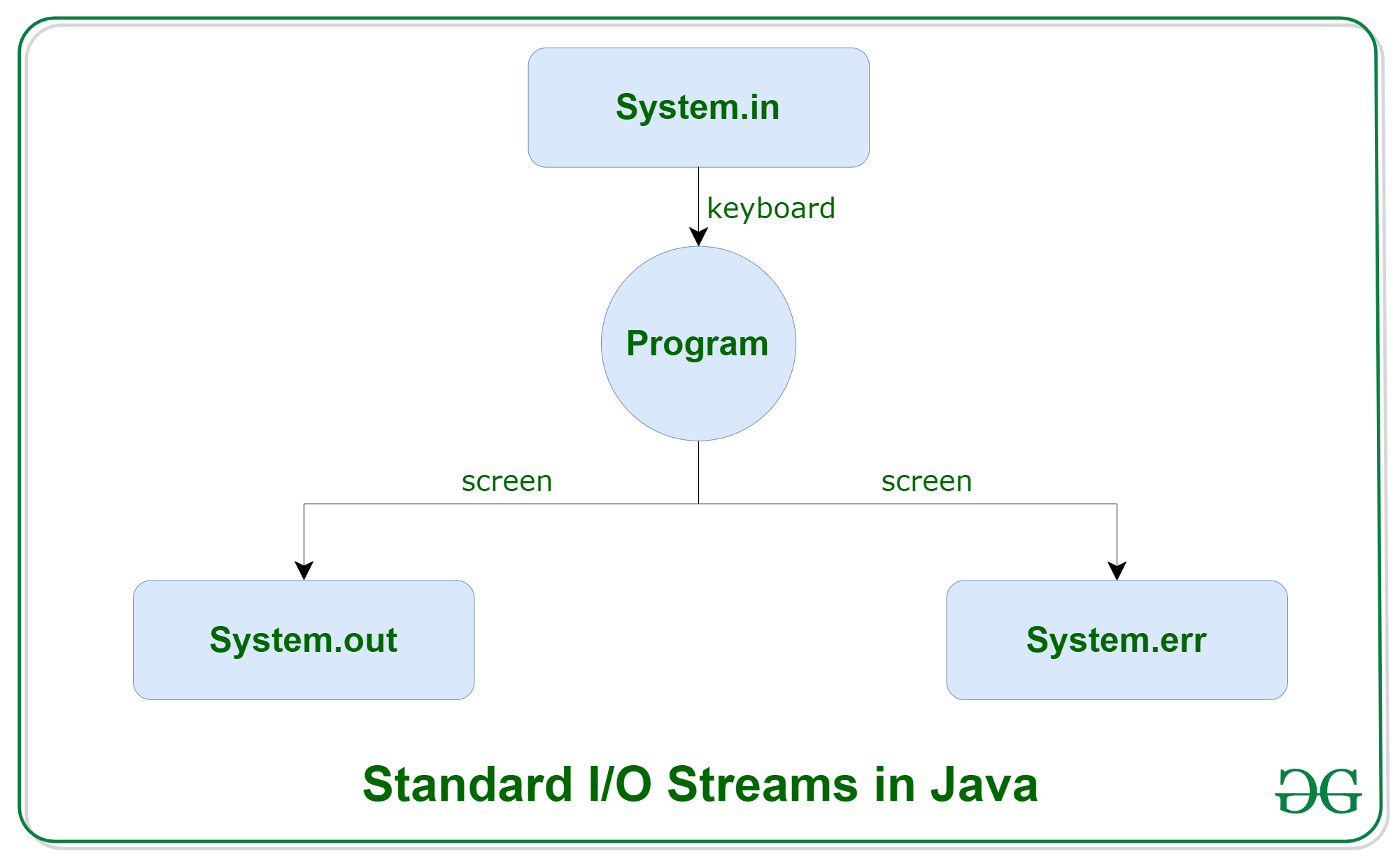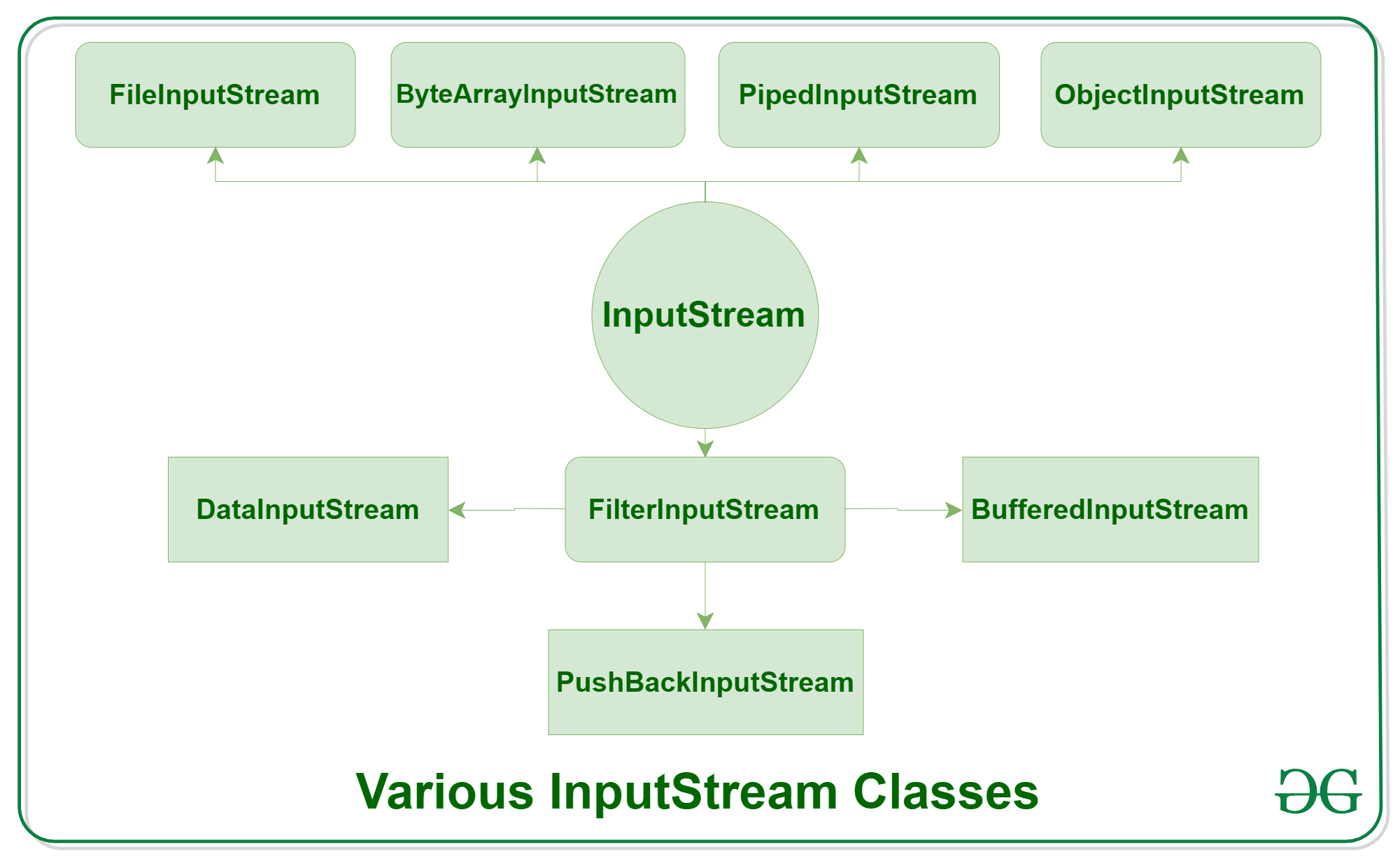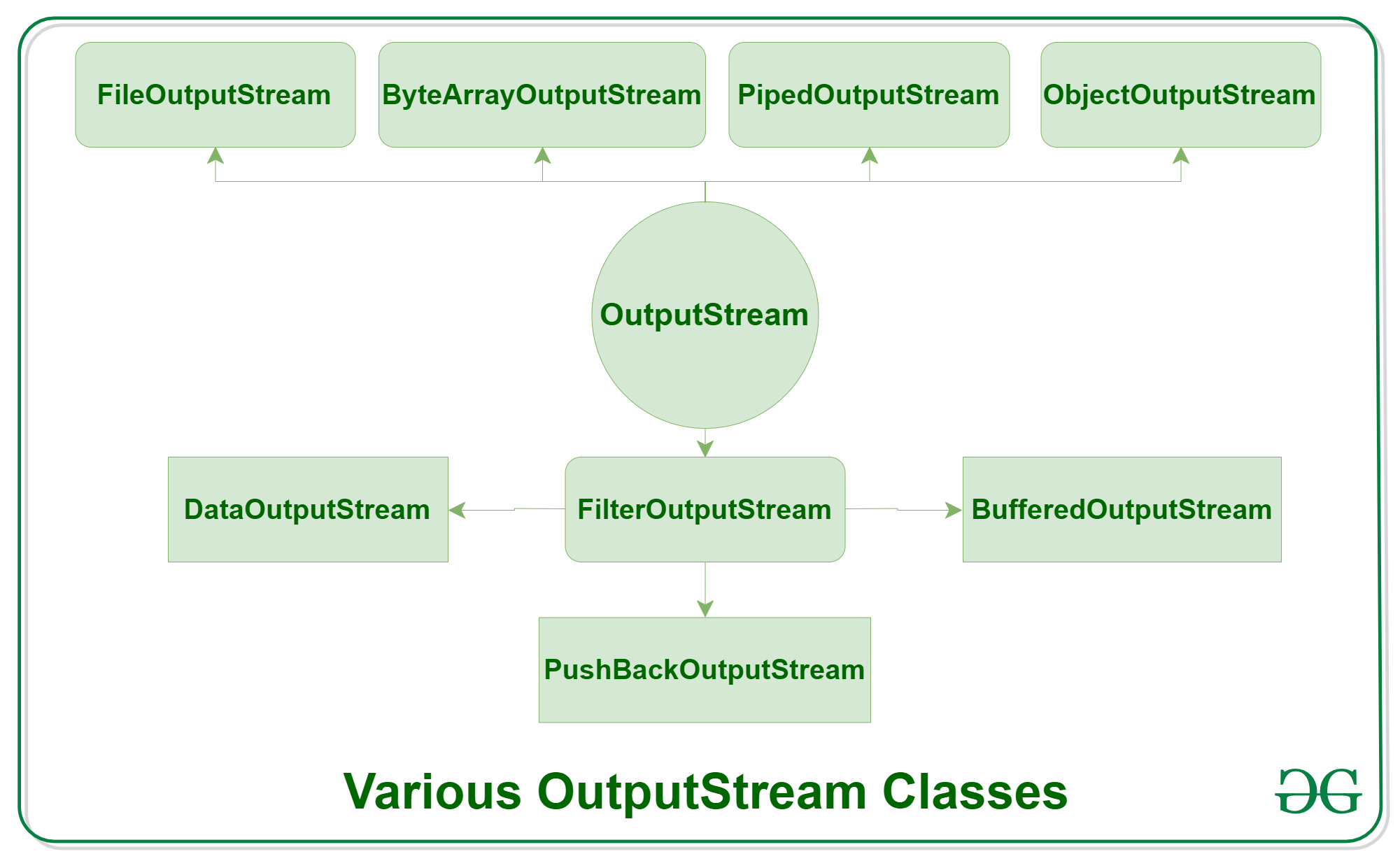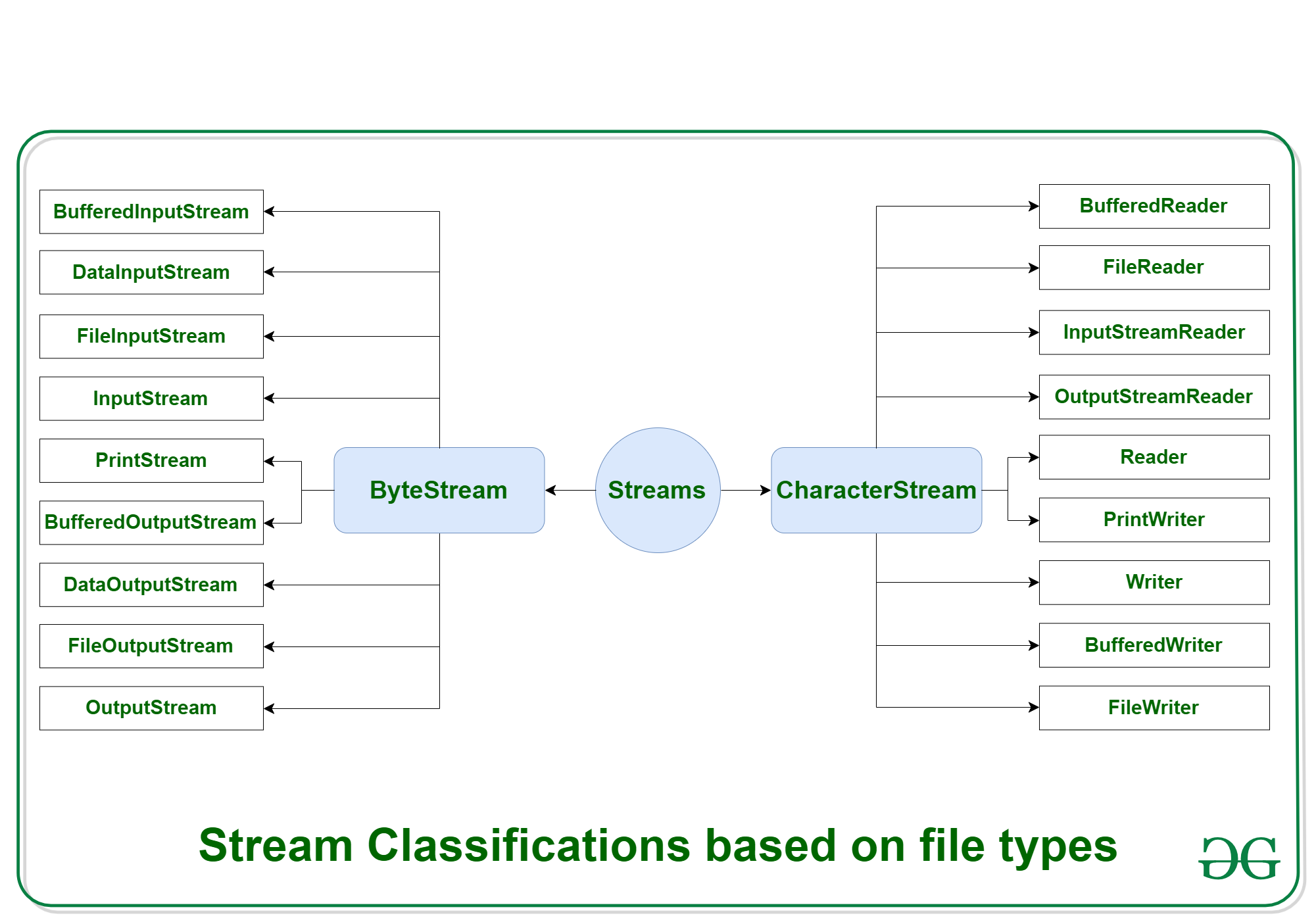# Java IO : Input-output in Java with Examples

Java brings various Streams with its I/O package that helps the user to perform all the input-output operations. These streams support all the types of objects, data-types, characters, files etc to fully execute the I/O operations.Before exploring various input and output streams lets look at 3 standard or default streams that Java has to provide which are also most common in use:1. System.in: This is the standard input stream that is used to read characters from the keyboard or any other standard input device.
2. System.out: This is the standard output stream that is used to produce the result of a program on an output device like the computer screen.

Here is a list of the various print functions that we use to output statements:

• print(): This method in Java is used to display a text on the console. This text is passed as the parameter to this method in the form of String. This method prints the text on the console and the cursor remains at the end of the text at the console. The next printing takes place from just here.
Syntax:

`System.out.print(parameter);`

Example:

 `// Java code to illustrate print() ` `import` `java.io.*; ` ` `  `class` `Demo_print { ` `    ``public` `static` `void` `main(String[] args) ` `    ``{ ` ` `  `        ``// using print() ` `        ``// all are printed in the ` `        ``// same line ` `        ``System.out.print(``"GfG! "``); ` `        ``System.out.print(``"GfG! "``); ` `        ``System.out.print(``"GfG! "``); ` `    ``} ` `} `

Output:

`GfG! GfG! GfG! `
• println(): This method in Java is also used to display a text on the console. It prints the text on the console and the cursor moves to the start of the next line at the console. The next printing takes place from the next line.
Syntax:

`System.out.println(parameter);`

Example:

 `// Java code to illustrate println() ` ` `  `import` `java.io.*; ` ` `  `class` `Demo_print { ` `    ``public` `static` `void` `main(String[] args) ` `    ``{ ` ` `  `        ``// using println() ` `        ``// all are printed in the ` `        ``// different line ` `        ``System.out.println(``"GfG! "``); ` `        ``System.out.println(``"GfG! "``); ` `        ``System.out.println(``"GfG! "``); ` `    ``} ` `} `

Output:

```GfG!
GfG!
GfG! ```
• printf(): This is the easiest of all methods as this is similar to printf in C. Note that System.out.print() and System.out.println() take a single argument, but printf() may take multiple arguments. This is used to format the output in Java.
Example:

 `// A Java program to demonstrate working of printf() in Java ` `class` `JavaFormatter1 { ` `    ``public` `static` `void` `main(String args[]) ` `    ``{ ` `        ``int` `x = ``100``; ` `        ``System.out.printf( ` `            ``"Printing simple"` `                ``+ ``" integer: x = %d\n"``, ` `            ``x); ` ` `  `        ``// this will print it upto ` `        ``// 2 decimal places ` `        ``System.out.printf( ` `            ``"Formatted with"` `                ``+ ``" precision: PI = %.2f\n"``, ` `            ``Math.PI); ` ` `  `        ``float` `n = ``5``.2f; ` ` `  `        ``// automatically appends zero ` `        ``// to the rightmost part of decimal ` `        ``System.out.printf( ` `            ``"Formatted to "` `                ``+ ``"specific width: n = %.4f\n"``, ` `            ``n); ` ` `  `        ``n = ``2324435``.3f; ` ` `  `        ``// here number is formatted from ` `        ``// right margin and occupies a ` `        ``// width of 20 characters ` `        ``System.out.printf( ` `            ``"Formatted to "` `                ``+ ``"right margin: n = %20.4f\n"``, ` `            ``n); ` `    ``} ` `} `

Output:

```Printing simple integer: x = 100
Formatted with precision: PI = 3.14
Formatted to specific width: n = 5.2000
Formatted to right margin: n =         2324435.2500```
3. System.err: This is the standard error stream that is used to output all the error data that a program might throw, on a computer screen or any standard output device.

This stream also uses all the 3 above-mentioned functions to output the error data:

• print()
• println()
• printf()
4. Example:

 `// Java code to illustrate standard ` `// input output streams ` ` `  `import` `java.io.*; ` `public` `class` `SimpleIO { ` ` `  `    ``public` `static` `void` `main(String args[]) ` `        ``throws` `IOException ` `    ``{ ` ` `  `        ``// InputStreamReader class to read input ` `        ``InputStreamReader inp = ``null``; ` ` `  `        ``// Storing the input in inp ` `        ``inp = ``new` `InputStreamReader(System.in); ` ` `  `        ``System.out.println(``"Enter characters, "` `                           ``+ ``" and '0' to quit."``); ` `        ``char` `c; ` `        ``do` `{ ` `            ``c = (``char``)inp.read(); ` `            ``System.out.println(c); ` `        ``} ``while` `(c != ``'0'``); ` `    ``} ` `} `

Input:

`GeeksforGeeks0`

Output:

```Enter characters, and '0' to quit.
G
e
e
k
s
f
o
r
G
e
e
k
s
0```

Types of Streams:

• Depending on the type of operations, streams can be divided into two primary classes:
1. Input Stream: These streams are used to read data that must be taken as an input from a source array or file or any peripheral device. For eg., FileInputStream, BufferedInputStream, ByteArrayInputStream etc.2. Output Stream: These streams are used to write data as outputs into an array or file or any output peripheral device. For eg., FileOutputStream, BufferedOutputStream, ByteArrayOutputStream etc.• Depending on the types of file, Streams can be divided into two primary classes which can be further divided into other classes as can be seen through the diagram below followed by the explanations.1. ByteStream: This is used to process data byte by byte (8 bits). Though it has many classes, the FileInputStream and the FileOutputStream are the most popular ones. The FileInputStream is used to read from the source and FileOutputStream is used to write to the destination. Here is the list of various ByteStream Classes:
Stream class Description
BufferedInputStream It is used for Buffered Input Stream.
DataInputStream It contains method for reading java standard datatypes.
FileInputStream This is used to reads from a file
InputStream This is an abstract class that describes stream input.
PrintStream This contains the most used print() and println() method
BufferedOutputStream This is used for Buffered Output Stream.
DataOutputStream This contains method for writing java standard data types.
FileOutputStream This is used to write to a file.
OutputStream This is an abstract class that describe stream output.

Example:

 `// Java Program illustrating the ` `// Byte Stream to copy ` `// contents of one file to another file. ` `import` `java.io.*; ` `public` `class` `BStream { ` `    ``public` `static` `void` `main( ` `        ``String[] args) ``throws` `IOException ` `    ``{ ` ` `  `        ``FileInputStream sourceStream = ``null``; ` `        ``FileOutputStream targetStream = ``null``; ` ` `  `        ``try` `{ ` `            ``sourceStream ` `                ``= ``new` `FileInputStream(``"sorcefile.txt"``); ` `            ``targetStream ` `                ``= ``new` `FileOutputStream(``"targetfile.txt"``); ` ` `  `            ``// Reading source file and writing ` `            ``// content to target file byte by byte ` `            ``int` `temp; ` `            ``while` `(( ` `                       ``temp = sourceStream.read()) ` `                   ``!= -``1``) ` `                ``targetStream.write((``byte``)temp); ` `        ``} ` `        ``finally` `{ ` `            ``if` `(sourceStream != ``null``) ` `                ``sourceStream.close(); ` `            ``if` `(targetStream != ``null``) ` `                ``targetStream.close(); ` `        ``} ` `    ``} ` `} `

Output:

`Shows contents of file test.txt `
2. CharacterStream: In Java, characters are stored using Unicode conventions (Refer this for details). Character stream automatically allows us to read/write data character by character. Though it has many classes, the FileReader and the FileWriter are the most popular ones. FileReader and FileWriter are character streams used to read from the source and write to the destination respectively. Here is the list of various CharacterStream Classes:
Stream class Description
BufferedReader It is used to handle buffered input stream.
InputStreamReader This input stream is used to translate byte to character.
OutputStreamReader This output stream is used to translate character to byte.
Reader This is an abstract class that define character stream input.
PrintWriter This contains the most used print() and println() method
Writer This is an abstract class that define character stream output.
BufferedWriter This is used to handle buffered output stream.
FileWriter This is used to output stream that writes to file.

Example:

 `// Java Program illustrating that ` `// we can read a file in a human-readable ` `// format using FileReader ` ` `  `// Accessing FileReader, FileWriter, ` `// and IOException ` `import` `java.io.*; ` `public` `class` `GfG { ` `    ``public` `static` `void` `main( ` `        ``String[] args) ``throws` `IOException ` `    ``{ ` `        ``FileReader sourceStream = ``null``; ` `        ``try` `{ ` `            ``sourceStream ` `                ``= ``new` `FileReader(``"test.txt"``); ` ` `  `            ``// Reading sourcefile and ` `            ``// writing content to target file ` `            ``// character by character. ` `            ``int` `temp; ` `            ``while` `(( ` `                       ``temp = sourceStream.read()) ` `                   ``!= -``1``) ` `                ``System.out.println((``char``)temp); ` `        ``} ` `        ``finally` `{ ` `            ``// Closing stream as no longer in use ` `            ``if` `(sourceStream != ``null``) ` `                ``sourceStream.close(); ` `        ``} ` `    ``} ` `} `

Whether you're preparing for your first job interview or aiming to upskill in this ever-evolving tech landscape, GeeksforGeeks Courses are your key to success. We provide top-quality content at affordable prices, all geared towards accelerating your growth in a time-bound manner. Join the millions we've already empowered, and we're here to do the same for you. Don't miss out - check it out now!

Previous
Next